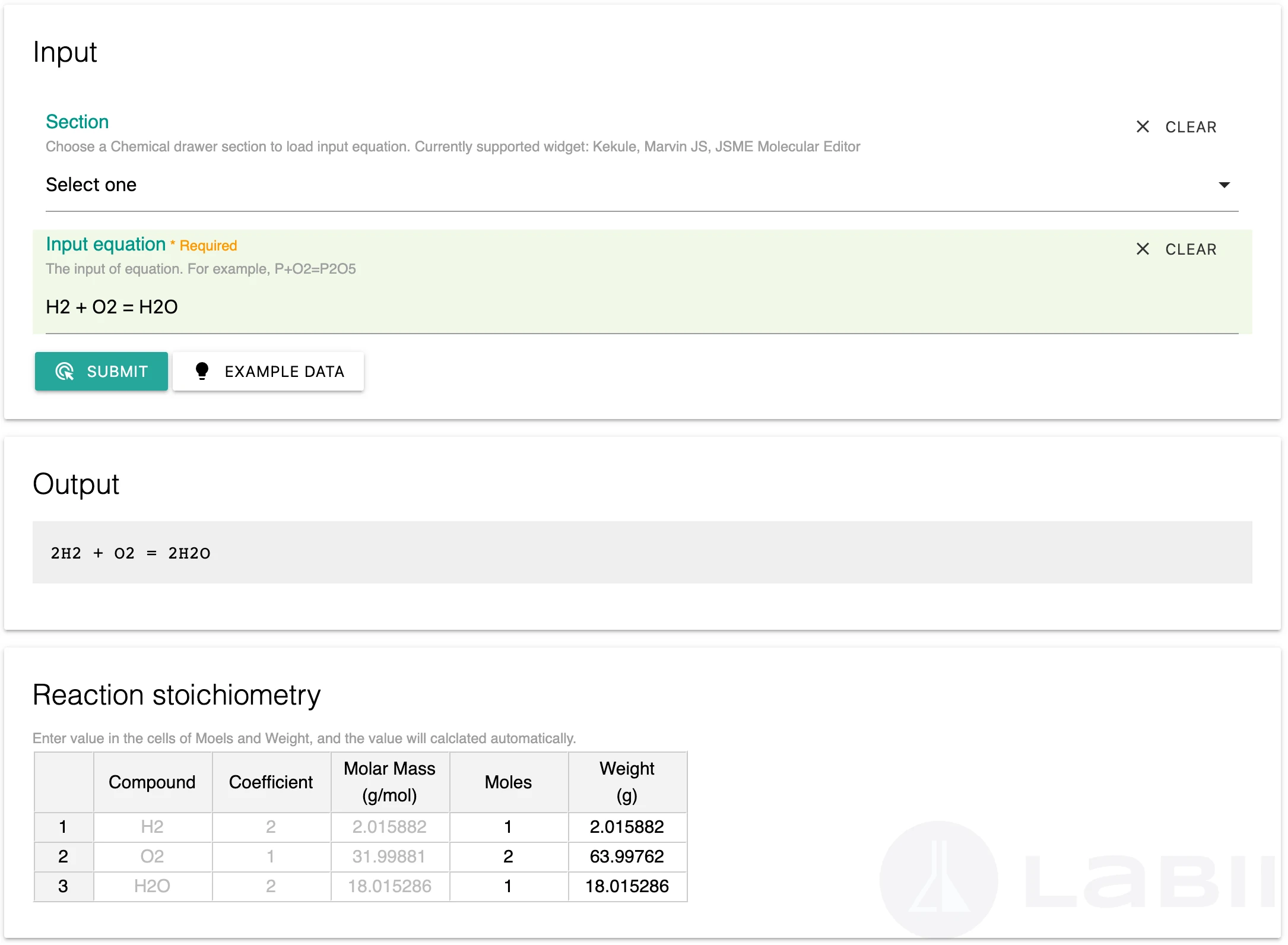# Chemical Reaction

Chemical reaction related widgets.

## Overview

A list of widgets has been developed in Labii to facilitate the chemical reactions.

## Chemical Equation Balancer

Chemical Equation is a form of describing a chemical reaction in which the name of each chemical substance will be replaced by its chemical symbol. In the Chemical Equation, the arrow direction represents the direction in which a reaction occurs.
A balanced equation is a chemical reaction equation in which the total charge and the number of atoms for each element in the reaction are the same for both the reactants and the components. In other words, the mass and charge on both sides of the reaction are equal.
Labii has developed a widget to perform the chemical equation balance and calculate the reaction stoichiometry.To use the chemical equation balancer:
1. 1.
Add a section with the widget Chemical Equation Balancer.
2. 2.
Enter an equation or select a section in the dropdown to load the equation directly from the chemical drawing widgets.
3. 3.
Click the Submit button.
4. 4.
The balanced equation will be displayed in the output section.

### Reaction stoichiometry

The stoichiometry can be generated by updating one value of the compound:
1. 1.
Enter a value into the cell of Moles or Weight, the rest of the values will be updated automatically.
2. 2.
Labii will save all values after the change.